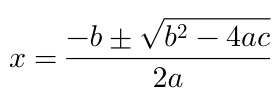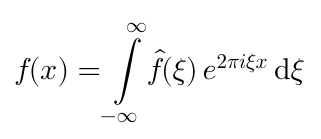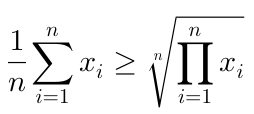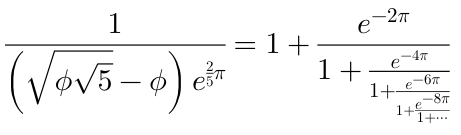# Swiftpack.co - kostub/iosMath as Swift Package

Swiftpack.co is a collection of thousands of indexed Swift packages. Search packages.
kostub/iosMath 0.9.5
Beautiful math equation rendering on iOS and MacOS
⭐️ 1,325
🕓 38 weeks ago
.package(url: "https://github.com/kostub/iosMath.git", from: "0.9.5")

# iosMath

iosMath is a library for displaying beautifully rendered math equations in iOS and MacOS applications. It typesets formulae written using the LaTeX in a UILabel equivalent class. It uses the same typesetting rules as LaTeX and so the equations are rendered exactly as LaTeX would render them.

It is similar to MathJax or KaTeX for the web but for native iOS or MacOS applications without having to use a UIWebView and Javascript. More importantly, it is significantly faster than using a UIWebView.

## Examples

Here are screenshots of some formulae that you could render with this library:The EXAMPLES.md file contains more examples.

## Requirements

iosMath works on iOS 6+ or MacOS 10.8+ and requires ARC to build. It depends on the following Apple frameworks:

• Foundation.framework
• CoreGraphics.framework
• QuartzCore.framework
• CoreText.framework

• UIKit.framework

• AppKit.framework

## Installation

### Cocoapods

iosMath is available through CocoaPods. To install it:

1. Add a entry for iosMath to your Podfile: pod 'iosMath'.
2. Install the pod by running pod install.

### Static library

You can also add iosMath as a static library to your project or workspace.

2. Open your project in Xcode, then drag and drop iosMath.xcodeproj onto your project or workspace (use the "Product Navigator view").
3. Select your target and go to the Build phases tab. In the Link Binary With Libraries section select the add button. On the sheet find and add libIosMath.a. You might also need to add iosMath to the Target Dependencies list.
4. Add the MathFontBundle to the list of Copy Bundle Resources.
5. Include IosMath wherever you need it with #import <IosMath/IosMath.h>.

## Usage

The library provides a class MTMathUILabel which is a UIView that supports rendering math equations. To display an equation simply create an MTMathUILabel as follows:

#import "MTMathUILabel.h"

MTMathUILabel* label = [[MTMathUILabel alloc] init];
label.latex = @"x = \\frac{-b \\pm \\sqrt{b^2-4ac}}{2a}";



Adding MTMathUILabel as a sub-view of your UIView as will render the quadratic formula example shown above.

### Included Features

This is a list of formula types that the library currently supports:

• Simple algebraic equations
• Fractions and continued fractions
• Exponents and subscripts
• Trigonometric formulae
• Square roots and n-th roots
• Calculus symbos - limits, derivatives, integrals
• Big operators (e.g. product, sum)
• Big delimiters (using \left and \right)
• Greek alphabet
• Combinatorics (\binom, \choose etc.)
• Geometry symbols (e.g. angle, congruence etc.)
• Ratios, proportions, percents
• Math spacing
• Overline and underline
• Math accents
• Matrices
• Equation alignment
• Change bold, roman, caligraphic and other font styles (\bf, \text, etc.)
• Most commonly used math symbols
• Colors

### Example

There is a sample app included in this project that shows how to use the app and the different equations that you can render. To run the sample app, clone the repository, and run pod install first. Then on iOS run the iosMathExample app. For MacOS run the MacOSMath app.

MTMathUILabel supports some advanced configuration options:

##### Math mode

You can change the mode of the MTMathUILabel between Display Mode (equivalent to  or \[ in LaTeX) and Text Mode (equivalent to \$ or \( in LaTeX). The default style is Display. To switch to Text simply:

label.labelMode = kMTMathUILabelModeText;

##### Text Alignment

The default alignment of the equations is left. This can be changed to center or right as follows:

label.textAlignment = kMTTextAlignmentCenter;

##### Font size

The default font-size is 20pt. You can change it as follows:

label.fontSize = 30;

##### Font

The default font is Latin Modern Math. This can be changed as:

label.font = [[MTFontManager fontManager] termesFontWithSize:20];


This project has 3 fonts bundled with it, but you can use any OTF math font.

##### Color

The default color of the rendered equation is black. You can change it to any other color as follows:

label.textColor = [UIColor redColor];


It is also possible to set different colors for different parts of the equation. Just access the displayList field and set the textColor on the underlying displays that you want to change the color of.

##### Custom Commands

You can define your own commands that are not already predefined. This is similar to macros is LaTeX. To define your own command use:

[MTMathAtomFactory addLatexSymbol:@"lcm"
value:[MTMathAtomFactory operatorWithName:@"lcm" limits:NO]];


This creates a \lcm command that can be used in the LaTeX.

##### Content Insets

The MTMathUILabel has contentInsets for finer control of placement of the equation in relation to the view.

If you need to set it you can do as follows:

label.contentInsets = UIEdgeInsetsMake(0, 10, 0, 20);

##### Error handling

If the LaTeX text given to MTMathUILabel is invalid or if it contains commands that aren't currently supported then an error message will be displayed instead of the label.

This error can be programmatically retrieved as label.error. If you prefer not to display anything then set:

label.displayErrorInline = NO;


## Future Enhancements

Note this is not a complete implementation of LaTeX math mode. There are some important pieces that are missing and will be included in future updates. This includes:

• Support for explicit big delimiters (bigl, bigr etc.)
• Addition of missing plain TeX commands

## Related Projects

For people looking for things beyond just rendering math, there are two related projects:

• MathEditor: A WYSIWYG editor for math equations on iOS.
• MathSolver: A library for solving math equations.